# Pressure in Fluids and Atmospheric Pressure

## Exercise 4(A)

#### Question 1

Define the term thrust. State it's S.I. unit.

Thrust is the force acting normally on a surface.

The S.I. unit of thrust is newton (N).

Thrust is a vector quantity.

#### Question 2

What is meant by pressure ? State its S.I. unit.

Pressure is the thrust per unit area of surface.

$Pressure = \dfrac{Thrust}{Area} \\[0.5em] \text{or} \\[0.5em] P = \dfrac{F}{A}$

Pressure is a scalar quantity.

The S.I. unit of pressure is newton per metre2 . This unit is named as pascal (symbol Pa).

#### Question 3

(a) What physical quantity is measured in bar ?

(b) How is the unit bar related to the S.I. unit pascal ?

(a) The physical quantity that is measured in bar is Pressure.

(b) The relation between bar and pascal is 1 bar = 105 pascal.

#### Question 4

Define one pascal (Pa), the S.I. unit of pressure.

One pascal is the pressure exerted on a surface of area 1 m2 by a force of 1 N acting normally on it.

#### Question 5

State whether thrust is a scalar or vector?

Thrust is a vector quantity. Its direction of application is normal to the surface.

#### Question 6

State whether pressure is a scalar or vector?

Pressure is a scalar quantity.

#### Question 7

Differentiate between thrust and pressure.

ThrustPressure
Thrust is the force acting normally on a surface.Pressure is the thrust per unit area of surface.
It is a vector quantityIt is a scalar quantity
The S.I. unit is newton(N)The S.I. is newton per metre2

#### Question 8

How does the pressure exerted by a thrust depend on the area of surface on which it acts ? Explain with a suitable example.

The pressure exerted by a thrust is inversely proportional to the area of surface on which it acts. Larger the area on which a given thrust acts, lesser is the pressure exerted by it.

Example — A brick of weight 4 kgf having dimensions 20 cm x 10 cm x 5 cm, exerts maximum pressure on ground when it is placed with it's longest side (20 cm) vertical, as shown in fig below, while it exerts minimum pressure when it is placed with it's shortest side (5 cm) vertical, even though the thrust is same in each case.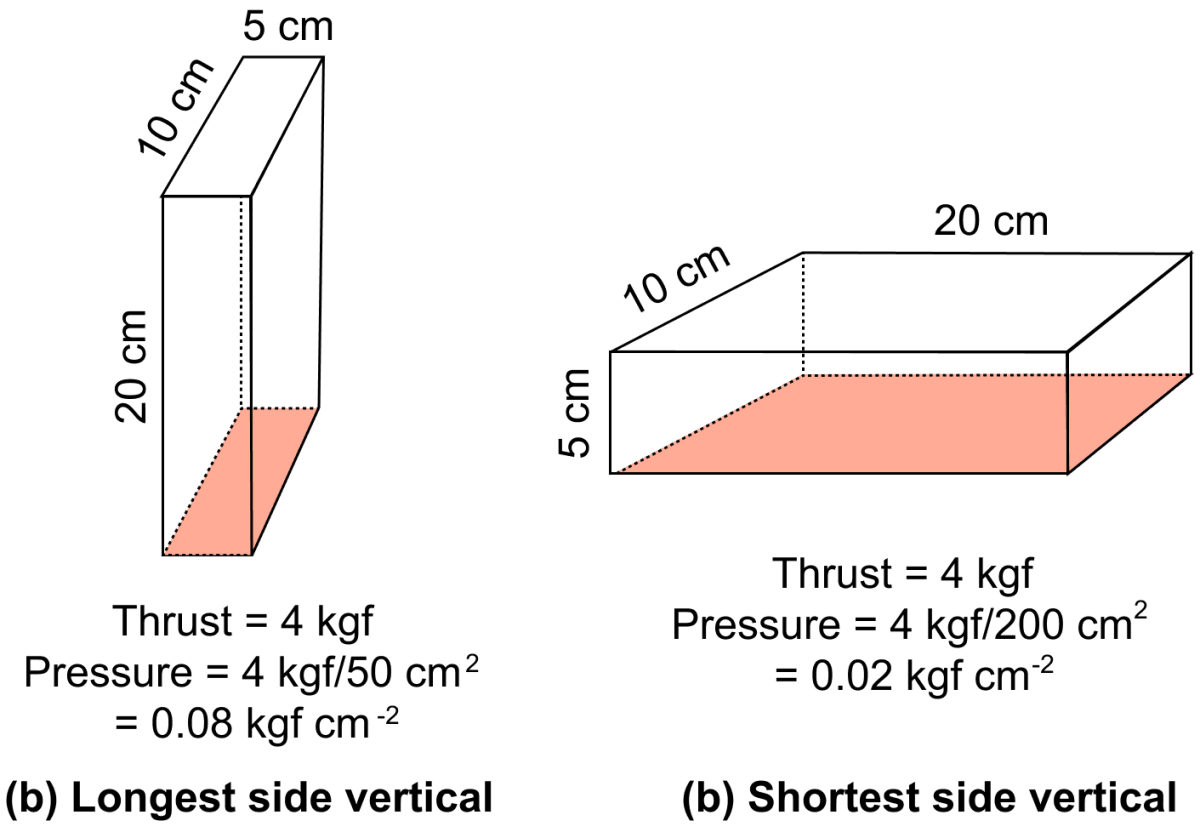#### Question 9

Why is the tip of an allpin made sharp?

The tip of an allpin is made sharp so that large pressure is exerted through the pointed end and that they can be driven into, with less effort.

#### Question 10

Explain the following —

(a) It is easier to cut with a sharp knife than with a blunt one.

(b) Sleepers are laid below the rails

(a) It is easier to cut with a sharp knife than with a blunt knife because in a sharp knife even a small thrust causes a great pressure at the edges and hence, cutting can be done with less effort.

(b) Sleepers are laid below the rails so that the pressure exerted by the iron nails on the ground becomes less.

#### Question 11

What is a fluid?

A substance which can flow is called a fluid. All liquids and gases are thus fluids.

#### Question 12

What do you mean by the term fluid pressure?

A fluid contained in a vessel exerts pressure at all points and in all directions due to it's weight and this pressure is called fluid pressure.

#### Question 13

How does the pressure exerted by a solid and a fluid differ ?

Both liquids and solids exert pressure due to it's weight, however, pressure exerted by a solid acts only on the surface on which it is placed i.e. at it's bottom, but pressure exerted by a fluid acts on the bottom as well as the walls of the container due to it's tendency to flow.

#### Question 14

Describe a simple experiment to demonstrate that a liquid enclosed in a vessel exerts pressure in all directions.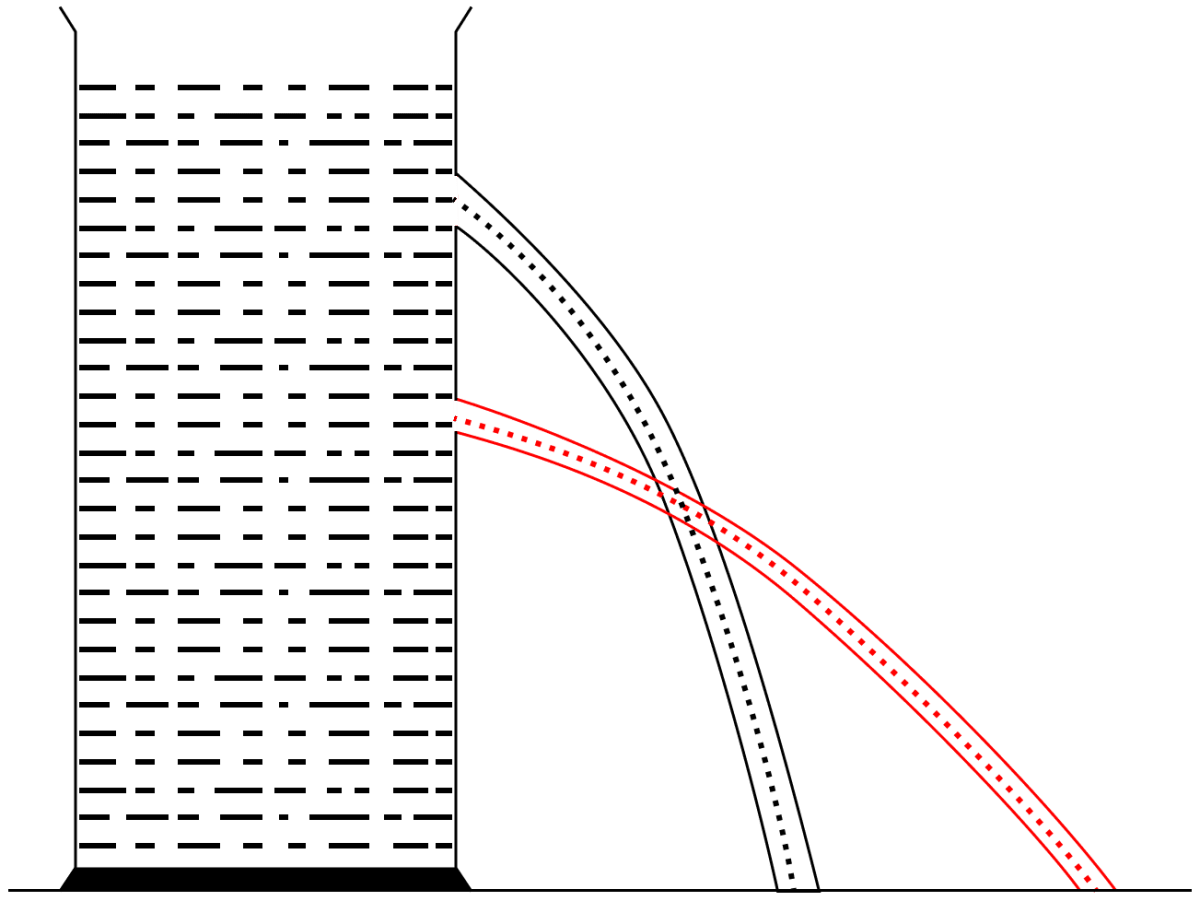Take a vessel filled with a liquid (say, water). Place it on a horizontal surface. Make several small holes in the wall of the vessel anywhere below the free surface of liquid. It is observed that liquid spurts out through each hole. This shows that the liquid exerts pressure at each point on the wall of the vessel.

#### Question 15

State three factors on which the pressure at a point in a liquid depends.

The three factors on which the pressure at a point in a liquid depends are —

1. depth of the point below the free surface (h),

2. density of liquid (ρ), and

3. acceleration due to gravity (g).

#### Question 16

Write an expression for the pressure at a point inside a liquid. Explain the meaning of the symbols used.

The pressure at a point inside a liquid at a depth h

= Atmospheric pressure + pressure due to liquid column

= P0 + hρg

where,

P0 = atmospheric pressure acting on the free surface of liquid

h = depth of the point below the free surface

ρ = the density of the fluid

g = acceleration due to gravity.

#### Question 17

Deduce an expression for the pressure at a depth inside a liquid.

Consider a vessel containing a liquid with density ρ. Let the liquid be stationary. In order to calculate pressure at a depth h, consider a horizontal circular surface PQ with area A at depth h below the free surface XY of the liquid as shown below.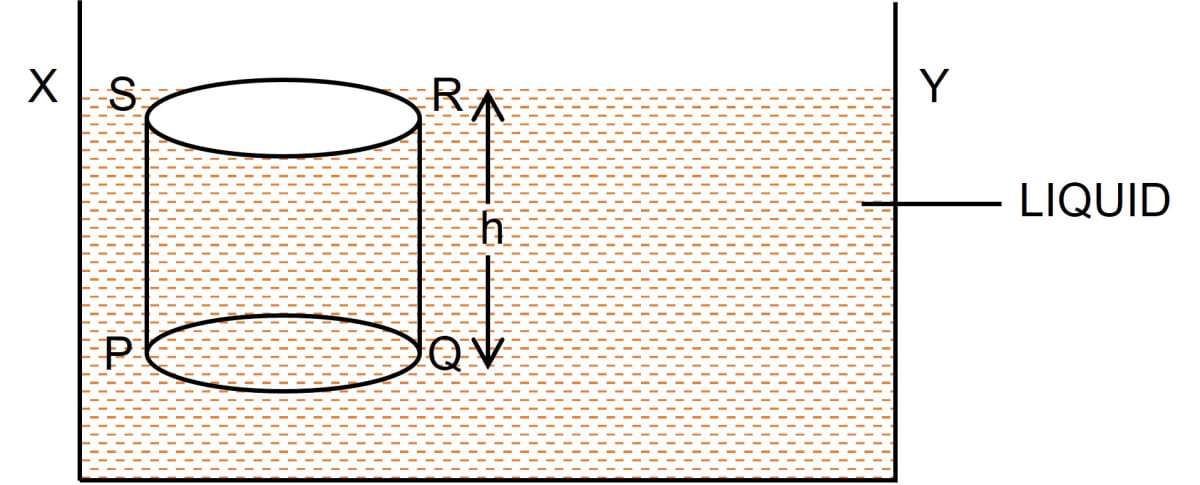The pressure on surface PQ will be due to the weight of the liquid column above the surface PQ, (i.e., the liquid contained in cylinder PQRS of height h with PQ as it's base and top face RS lying on the free surface XY of the liquid).

Thrust exerted on the surface PQ

= Weight of the liquid column PQRS

= Volume of liquid column PQRS x density x g

= (Area of base PQ x height) x density x g

= (A x h) ρ x g = A h ρ g

This thrust is exerted on the surface PQ of area A. Therefore, pressure

P = $\dfrac{\text{Thrust on surface}}{\text{Area of surface}}$ = $\dfrac{\text{A h ρ g}}{\text{A}}$ = h ρ g

Hence, Pressure = depth x density of liquid x acceleration due to gravity = h ρ g

#### Question 18

How does the pressure at a certain depth in sea water differ from that at the same depth in river water ? Explain your answer.

The pressure at a certain depth in sea water is more than the same depth in river water because the density of sea water is more than the density of river water.

According to the formula,

Pressure = depth x density of liquid x acceleration due to gravity = h ρ g

Therefore, when density of a liquid is more then the pressure exerted is also more as density and pressure are directly proportional.

#### Question 19

Pressure at free surface of a water lake is P1, while at a point at depth h below it's free surface is P2. (a) How are P1 and P2 related ? (b) Which is more P1 or P2 ?

(a) As we know,

Total pressure in a liquid at a depth h is P2

= atmospheric pressure acting on the surface (P1) + pressure due to liquid column (h ρ g)

= P1 + h ρ g

Hence,

P2 = P1 + h ρ g

(b) From the above expression we observe that,

P2 > P1

#### Question 20

Explain why a gas bubble released at the bottom of a lake grows in size as it rises to the surface of lake.

It is noticed that as the gas bubble formed at the bottom of the lake rises, it grows in size. The reason is that when the bubble is at the bottom of the lake, total pressure exerted on it is the sum of the atmospheric pressure and the pressure due to the water column above it.

As the gas bubble rises, due to decrease in depth, the pressure due to water column decreases, so the total pressure on the bubble decreases.

According to Boyle's law, the volume of a gas is inversely proportional to the pressure on it. Therefore, the volume of bubble increases due to the decrease in pressure, i.e., the bubble grows in size.

When the bubble reaches the surface of liquid, total pressure exerted on it becomes minimum, just equal to the atmospheric pressure and so the size of bubble when touching the surface becomes maximum.

#### Question 21

A dam has broader walls at the bottom than at the top. Explain.

A dam has broader walls at the bottom than at the top as the pressure exerted by the liquid increases with it's depth. Thus, as depth increases, more and more pressure is exerted by water on the walls of the dam.

A thicker wall is required to withstand a greater pressure, therefore, the wall of a dam is made with thickness increasing towards the base.

In the figure given below, the increasing length of arrows in water represents the increasing pressure on the wall of the dam towards the bottom.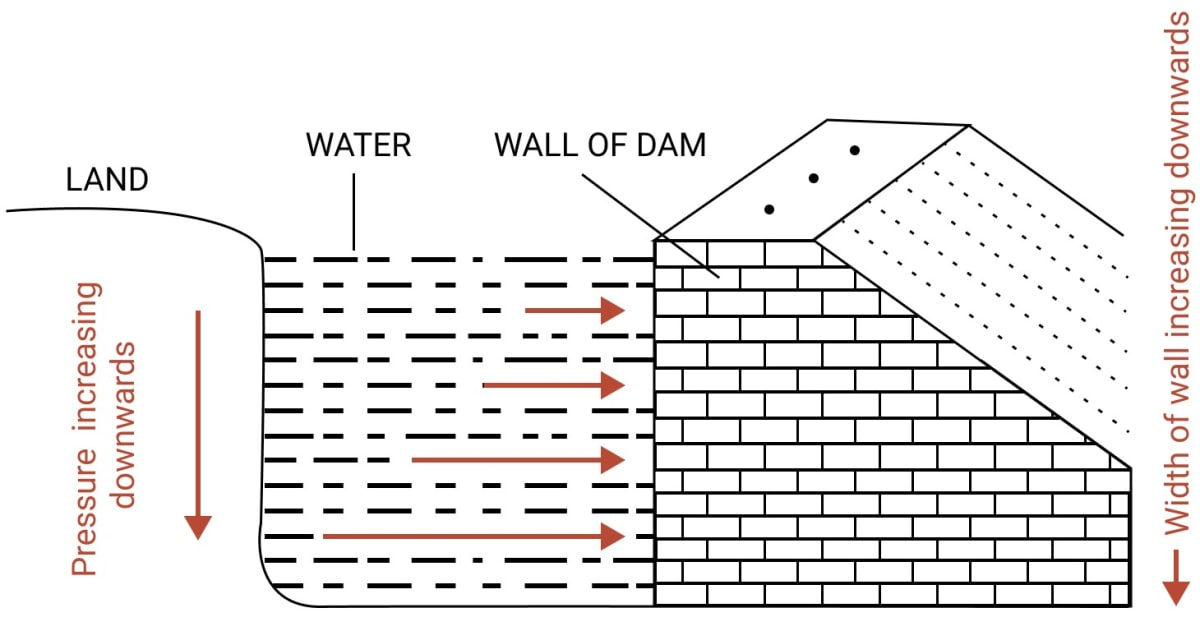#### Question 22

Why do sea divers need special protective suit ?

Sea divers need special protective suit to wear because in deep sea, the total pressure exerted on the diver's body is much more than his blood pressure. To withstand it, they need to wear a special protective suit, made from glass reinforced plastic or cast aluminium. The pressure inside the suit is maintained at one atmosphere.

#### Question 23

State the laws of liquid pressure.

The laws of liquid pressure are —

1. Inside the liquid, pressure increases with the increase in depth from it's free surface.

2. In a stationary liquid, pressure is same at all points on a horizontal plane.

3. Pressure is same in all directions about a point inside the liquid.

4. Pressure at same depth is different in different liquids. It increases with the increase in density of liquid.

5. A liquid seeks it's own level.

#### Question 24

A tall vertical cylinder filled with water is kept on a horizontal table top. Two small holes A and B are made on the wall of the cylinder, A near the middle and B just below the free surface of water. State and explain your observation.

We observe that the throw of the liquid from hole A is more than the B i.e., the liquid reaches to a greater distance on the horizontal surface from hole A than hole B. This shows that liquid pressure at a point increases with the increase of depth from it's free surface.

#### Question 25

How does the liquid pressure on a diver change if —

(i) the diver moves to the greater depth, and

(ii) the diver moves horizontally ?

(i) When the diver moves to a greater depth the liquid pressure increases as liquid pressure at a point increases with the increase of depth from it's free surface.

(ii) When the diver moves horizontally, the liquid pressure remains unchanged as inside a liquid, pressure is same at all points on a horizontal plane.

#### Question 26

State Pascal's law of transmission of pressure.

Pascal's law states that the pressure exerted anywhere in a confined liquid is transmitted equally and undiminished in all directions throughout the liquid.

#### Question 27

Name two applications of Pascal's law.

Applications of Pascal's law are —

1. Hydraulic brakes
2. Hydraulic jack

#### Question 28

Explain the principle of a hydraulic machine. Name two devices which work on this principle.

The principle of hydraulic machine is that a small force applied on a smaller piston is transmitted to produce a large force on the bigger piston.

Two devices that work on this principle are —

1. Hydraulic brakes
2. Hydraulic jack

#### Question 29

Name and state the principle on which a hydraulic press works. Write one use of the hydraulic press.

A hydraulic press works on the principle of Pascal's law.

Principle — When a force F1 is applied on the piston A, it exerts a pressure on liquid contained in the cylinder P. According to Pascal's law, this pressure is transmitted through liquid in tube R to the piston B of the other cylinder Q due to which the piston B tends to move upwards.

Since, the area of cross section of cylinder P is less than that of the cylinder Q, therefore by applying a small force on the piston A, we can lift a large weight kept on the piston B.

When no weight is placed on the piston B, it rises up against a fixed roof with a force F2 (F2 > F1). If a bale of cotton is kept on the press plunger B, it gets compressed.

Use of hydraulic press — It is used for pressing cotton bales and goods such as books, quilts etc.

#### Question 30

The diagram in figure shows a device which makes use of the principle of transmission of pressure.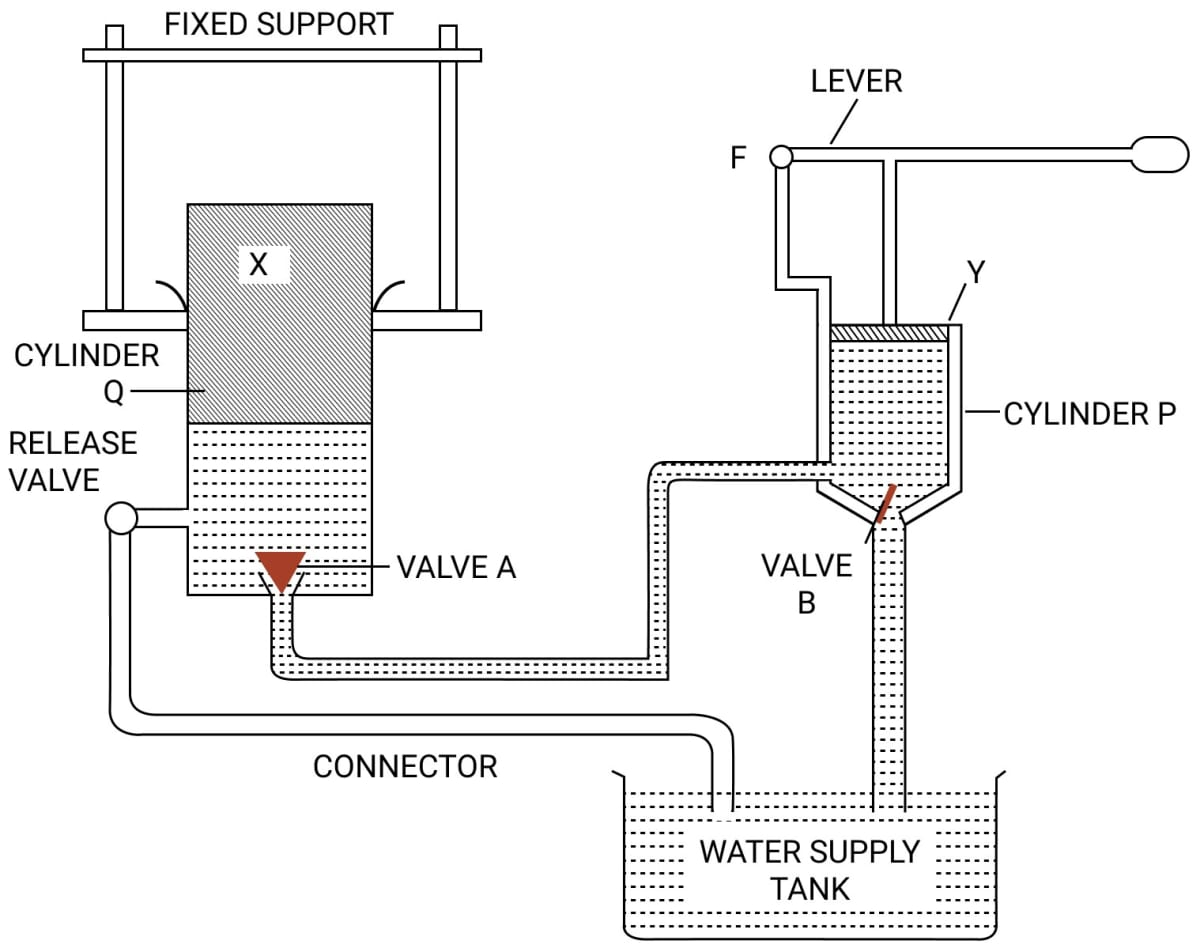(i) Name the parts labelled by the letters X and Y.

(ii) Describe what happens to the valves A and B and to the quantity of water in the two cylinders when the lever arm is moved down.

(iii) Give reasons for what happens to the valves A and B in part (ii).

(iv) What happens when the release valve is opened ?

(v) What happens to the valve B in cylinder P when the lever arm is moved up ?

(vi) Give a reason for your answer in part (v)

(vii) State one use of the above device.

(i) The parts are —

X → Press plunger

Y → Pump plunger

(ii) When the lever arm is moved down, the valve B closes and the valve A opens, so water from cylinder P is forced into the cylinder Q.

(iii) When the lever arm is moved down, the valve B closes due to an increase in pressure in cylinder P. Now pressure from cylinder P is transmitted to the connecting pipe. As the pressure in connecting pipe becomes greater than the cylinder Q, the valve A opens, so water from cylinder P is forced into the cylinder Q.

(iv) When the release valve is opened the ram (or press) plunger Q gets lowered and water of the cylinder Q runs out into the reservoir.

(v) When the lever arm is moved up the valve B opens upwards.

(vi) When the lever arm is moved up the valve B opens upward because the pressure in cylinder P decreases.

(vii) An hydraulic press is used for pressing cotton bales and goods like quilts, books, etc.

#### Question 31

Draw a simple diagram of a hydraulic jack and explain it's working.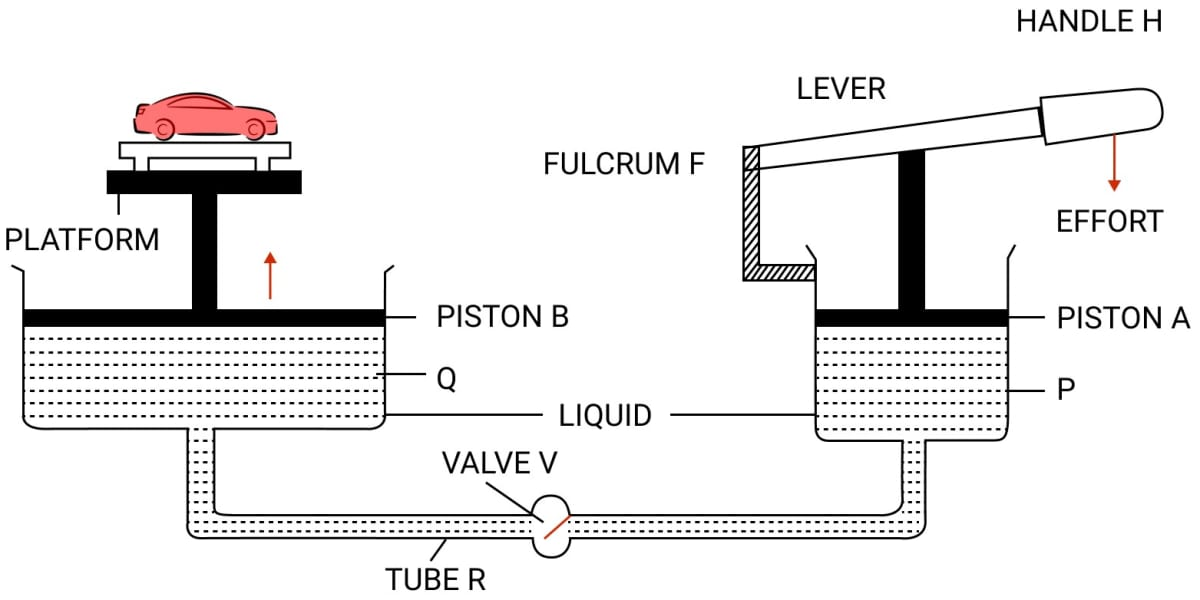When handle H of lever is pressed down by applying an effort, the valve V opens because of increase in pressure in the cylinder P. The liquid runs out from the cylinder P to the cylinder Q.

As a result, the piston B rises up and it raises the car placed on the platform. When the car reaches the desired height, the handle H of lever is no longer pressed.

The valve V gets closed (since the pressure on either side of the valve becomes same) so that the liquid may not run back from the cylinder Q to the cylinder P.

#### Question 32

Explain the working of a hydraulic brake with a simple labelled diagram.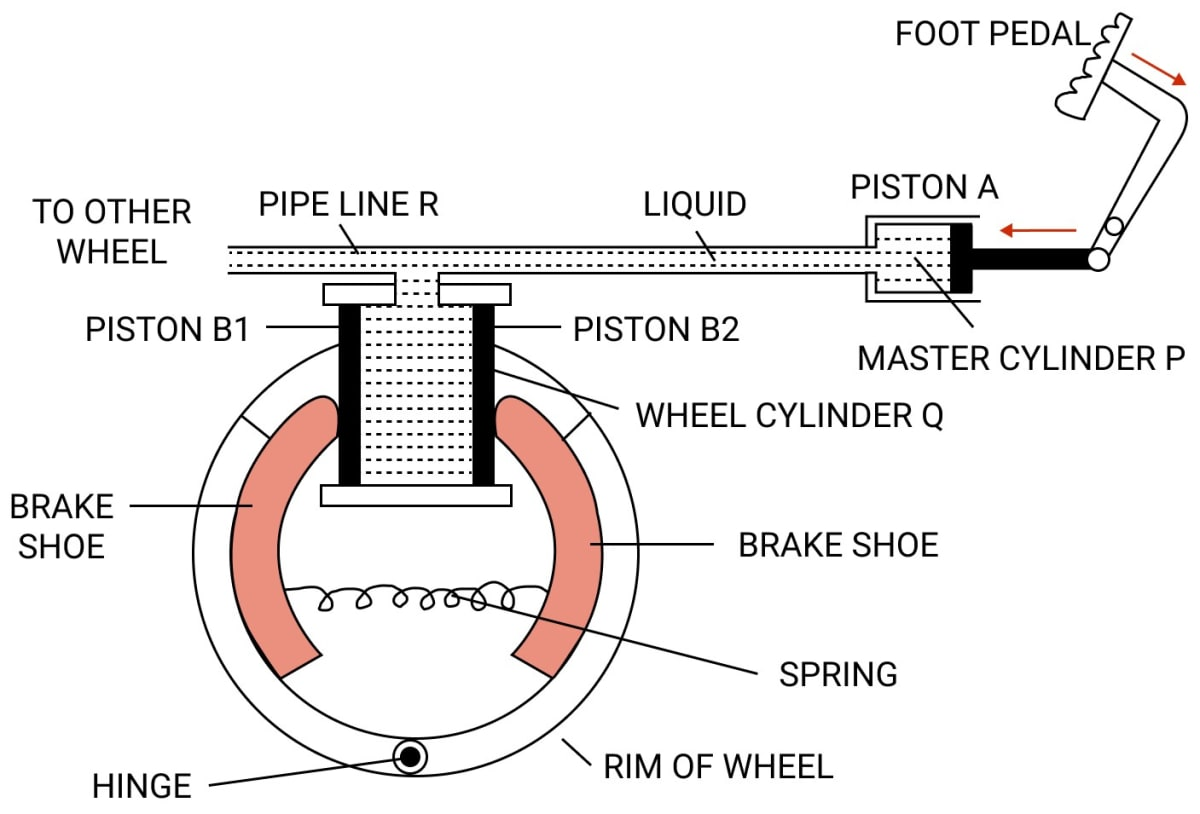To apply brakes, the foot pedal is pressed due to which pressure is exerted on the liquid in the master cylinder P, so liquid runs out from the master cylinder P to the wheel cylinder Q.

As a result, the pressure is equally transmitted and undiminished through the liquid to the pistons B1 and B2 of the wheel cylinder Q. Therefore, the pistons B1 and B2 gets pushed outwards and brake shoes gets pressed against the rim of the wheel due to which the motion of the wheel retards.

Since, the area of cross section of piston A in the master cylinder P is less than that in the wheel cylinder Q, a small force applied at the foot pedal produces a large force on the pistons B1 and B2 of the wheel cylinder Q.

This is the force responsible for retarding the vehicle. It should be noted that due to transmission of pressure through liquid, equal pressure is exerted on all wheels of the vehicle connected to the pipe line R.

On releasing the pressure on the pedal, the spring pulls the brake shoes to it's original position and forces the piston's B1 and B2 to return back into the wheel cylinder Q. As a result the liquid runs back from the wheel cylinder Q to the master cylinder P and thus the brakes gets released.

#### Question 33

Complete the following sentences —

(a) Pressure at a depth h in a liquid of density ρ is ___________ .

(b) Pressure is ________ in all directions about a point in a liquid.

(c) Pressure at all points at the same depth is _________.

(d) Pressure at a point inside a liquid is __________ to it's depth.

(e) Pressure of a liquid at a given depth is _______ to the density of liquid.

(a) Pressure at a depth h in a liquid of density ρ is h ρ g.

(b) Pressure is same in all directions about a point in a liquid.

(c) Pressure at all points at the same depth is same.

(d) Pressure at a point inside a liquid is directly proportional to its depth.

(e) Pressure of a liquid at a given depth is directly proportional to the density of liquid.

## Multiple Choice Type

#### Question 1

The S.I. unit of pressure is:

1. N cm-2
2. Pa ✓
3. N
4. N m2

Pressure is the thrust per unit area of surface.

$Pressure = \dfrac{Thrust}{Area} \\[0.5em] \text{or} \\[0.5em] P = \dfrac{F}{A}$

Pressure is a scalar quantity.

The S.I. unit of pressure is Pascal

One pascal is the pressure exerted on a surface of area 1 m2 by a force of 1 N that acts normally on it.

#### Question 2

The pressure inside a liquid of density ρ at a depth h is:

1. hρg ✓
2. h/ρg
3. hρ/g

The three factors on which the pressure at a point in a liquid depends are —

1. depth of the point below the free surface (h)

2. density of liquid (ρ) and

3. acceleration due to gravity (g)

Pressure = hρg

#### Question 3

The pressure P1 at a certain depth in river water and P2 at the same depth in sea water are related as:

1. P1> P2
2. P1 = P2
3. P1 < P2
4. P1 - P2 = atmospheric pressure

As we know,

Total pressure in a liquid at a depth h is P2

= atmospheric pressure acting on the surface (P1) + pressure due to liquid column (hρg)

= P1 + hρg

Hence,

P2 = P1 + h ρ g

P1 < P2

#### Question 4

The pressure P1 at the top of a dam and P2 at a depth h from the top inside water (density ρ) are related as:

1. P1 > P2
2. P1 = P2
3. P1 - P2 = hρg
4. P2 – P1 = hρg ✓

The pressure at a point inside a liquid at a depth h is P2

P2 = pressure at the top of a dam (P1) + pressure due to liquid column

= P1 + hρg

So, P2 = P1 + hρg

Hence,

P2 - P1 = hρg

## Numericals

#### Question 1

A hammer exerts a force of 1.5 N on each of the two nails A and B. The area of cross section of tip of nail A is 2 mm2 while that of nail B is 6 mm2 . Calculate pressure on each nail in pascal.

As we know,

Pressure (P) = $\dfrac{\text {Thrust (F)}}{\text {Area (A)}}$

Given,

F = 1.5 N

A = 2 mm2

Converting 2 mm2 in metre2

As 1 mm = $\dfrac{1}{1000}$ m

So, 1 mm2 = (1) mm x (1) mm

= $(\dfrac{1}{1000}) m \times (\dfrac{1}{1000}) m$

= 1 x 10 -6 m2

Hence,

2 mm2 = 2 x 10-6 m2

AA = 2 x 10 -6 m2

AB = 6 x 10 -6 m2

Substituting the values in the formula above, we get,

Pressure on A

$P = \dfrac{1.5}{2 \times 10^{-6}} \\[0.5em] P = 0.75 \times 10^{6} \\[0.5em] \Rightarrow P = 7.5 \times 10^{5} Pa \\[0.5em]$

Hence, Pressure on A = 7.5 x 105 Pa

Pressure on B

$P = \dfrac{1.5}{6 \times 10^{-6}} \\[0.5em] P = 0.25 \times 10^{6} \\[0.5em] \Rightarrow P = 2.5 \times 10^{5} Pa \\[0.5em]$

Hence, Pressure on B = 2.5 x 105 Pa

#### Question 2

A block of iron of mass 7.5 kg and of dimensions 12 cm x 8 cm x 10 cm is kept on a table top on it's base of side 12 cm x 8 cm. Calculate (a) thrust and (b) pressure exerted on the table top. Take 1 kgf = 10 N.

As we know,

(a) Force (F) = mass (m) x acceleration due to gravity (g)

Given,

m = 7.5 kg

1 kgf = 10 N

Substituting the values in the formula above we get,

$F = 7.5 \times 10 \\[0.5em] \Rightarrow F = 75 N$

Hence, F = 75 N

(b) As we know,

Pressure (P) = $\dfrac{\text {Thrust (F)}}{\text {Area (A)}}$

Given,

Area of the base = 12 x 8 = 96 cm2

Converting cm2 into m2

100 cm = 1 m

So, 100 cm x 100 cm = 1 m2

Hence, 96 cm2 = $\dfrac{1 \times 96}{10000}$

Therefore, A = 0.0096 m 2

Substituting the values in the formula above, we get,

$P = \dfrac{75}{0.0096} \\[0.5em] \Rightarrow P = 7812.5.5 Pa \\[0.5em]$

Hence,

Pressure = 7812.5 Pa

#### Question 3

A vessel contains water up to a height of 1.5 m. Taking the density of water 10 3 kg m -3, acceleration due to gravity 9.8 m s -2 and area of base vessel 100 cm 2, calculate (a) the pressure and (b) the thrust, at the base of vessel.

(a) As we know,

Pressure due to water column of height h = h ρ g

Given,

h = 1.5 m

ρ = 1000 kg m -3

g = 9.8 m s 2

Substituting the values in the formula above, we get,

$P = 1.5 \times 1000 \times 9.8 \\[0.5em] P = 14.7 \times 10^{3} \text {N m}^{-2} \\[0.5em]$

Hence, pressure = 1.47 x 104 N m-2

(b) As we know,

Pressure (P) = $\dfrac{\text {Thrust (F)}}{\text {Area (A)}}$

Given,

A = 100 cm 2

Converting cm 2 into m 2

100 cm = 1 m

So, 100 cm x 100 cm = 1 m 2

Hence, 100 cm 2 = $\dfrac{1 \times 100}{10000}$

Therefore, A = 10-2 m 2

Substituting the values in the formula above, we get,

$1.47 \times 10^{4} = \dfrac{\text {Thrust (F)}}{10^{-2}} \\[0.5em] \Rightarrow \text {Thrust (F)} = 1.47 \times 10^{2} \\[0.5em] \Rightarrow \text {Thrust (F)} = 147 N \\[0.5em]$

Hence, Thrust = 147 N

#### Question 4

The area of base of a cylindrical vessel is 300 cm 2. Water (density = 1000 kg m -3) is poured into it up to a depth of 6 cm. Calculate (a) the pressure and (b) the thrust of water on the base. (g = 10 m s-2).

(a) As we know,

Pressure due to water column of height h = hρg

Given,

ρ = 1000 kg m -3

g = 10 m s-2

h = 6 cm

Converting cm to m

100 cm = 1 m

So, 6 cm = $\dfrac{1}{100}$ x 6 = 0.06 m

Hence, h = 0.06 m

Substituting the values in the formula above we get,

$P = 0.06 \times 1000 \times 10 \\[0.5em] P = 600 \text {Pa} \\[0.5em]$

Hence, Pressure = 600 Pa

(b) As we know,

Pressure (P) = $\dfrac{\text {Thrust (F)}}{\text {Area (A)}}$

A = 300 cm 2

Converting cm 2 into m 2

100 cm = 1 m

So, 100 cm x 100 cm = 1 m 2

Hence, 300 cm 2 = $\dfrac{1 \times 300}{10000}$

Therefore, A = 3 x 10-2 m 2

Substituting the values in the formula we get,

$600 = \dfrac{\text {Thrust (F)}}{3 \times 10^{-2}} \\[0.5em] \Rightarrow \text {Thrust (F)} = 600 \times 3 \times 10^{-2}\\[0.5em] \Rightarrow \text {Thrust (F)} = 18 N \\[0.5em]$

Hence, thrust = 18 N

#### Question 5

(a) Calculate the height of a water column which will exert on it's base the same pressure as the 70 cm column of mercury. Density of mercury is 13.6 g cm-3.

(b) Will the height of the water column in part (a) change if the cross section of the water column is made wider ?

(a) As we know,

Pressure due to water column of height h = hρg

and

Pascal's law, states that the pressure exerted anywhere in a confined liquid is transmitted equally and undiminished in all directions throughout the liquid.

Hence,

Pressure due to water column = Pressure due to mercury column

Hence,

hw ρw g = hm ρm g

Given,

hm = 70 cm

ρm = 13.6 g cm-3

ρw = 1 g cm-3

From the above formula, we get,

hw = $\dfrac{h_m ρ_m}{ρ_w}$

Substituting the values, we get,

$h_w = (\dfrac{13.6}{1}) \times 70 \\[0.5em] \Rightarrow h_w = 952 \text{cm} \\[0.5em] \Rightarrow h_w = 9.52 \text{m} \\[0.5em]$

Hence, height of a water column = 9.52 m

(b) No, if the cross section of the water column is made wider, the height of the water column will be unaffected.

#### Question 6

The pressure of water on the ground floor is 40,000 Pa and on the first floor is 10,000 Pa. Find the height of the first floor.
(Take : density of water = 1000 kg m-3, g = 10 m s-2)

(i) As we know,

Pressure due to water column of height h = h ρ g

Given,

density of water = 1000 kg m -3

g = 10 m s-2

Pw on ground floor = 40,000 Pa

Pw on first floor Pwf = 10,000 Pa

In order to know the height of the first floor, let us calculate the difference in pressure

P = Pwg - Pwf = 40,000 – 10,000 = 30,000 Pa

Substituting the values in the formula,

$P = h ρ g \\[0.5em] 30,000 = 1000 \times 10 \times h \\[0.5em] h = 3 m \\[0.5em]$

Hence, the height of the first floor = 3 m.

#### Question 7

A simple U tube contains mercury to the same level in both of it's arms. If water is poured to a height of 13.6 cm in one arm, how much will be the rise in mercury level in the other arm ?

Given: density of mercury = 13.6 x 103 kg m-3 and density of water = 103 kg m-3

Given,

ρm = 13.6 x 103 kg m -3

ρw = 103 kg m -3

hw = 13.6 cm

hm = x cm

As we know,

Pascal's law, states that the pressure exerted anywhere in a confined liquid is transmitted equally and undiminished in all directions throughout the liquid.

Hence,

Pressure in the water column = pressure in the mercury column

Therefore, PA = PB

Now,

PA due to liquid of height 13.6 cm is

PA = h ρ g = (13.6) x (103) x (g)    [Equation 1]

PB due to mercury of height x is

PB = (𝑥) x (13.6 x 103) x (g)    [Equation 2]

Equating 1 and 2 we get,

(13.6) x (103) x (g) = (𝑥) x (13.6 x 103) x (g)

Hence, the rise in mercury level = 1 cm

#### Question 8

In a hydraulic machine, a force of 2 N is applied on the piston of area of cross section 10 cm 2. What force is obtained on it's piston of area of cross section 100 cm 2?

As we know,

Pressure (P) = $\dfrac{\text {Thrust (F)}}{\text {Area (A)}}$

Given,

A = 10 cm 2

Converting cm 2 into m 2

100 cm = 1 m

So, 100 cm x 100 cm = 1 m 2

Hence, 10 cm 2 = $\dfrac{1 \times 10}{10000}$

Therefore, A = 10-3 m 2

F = 2 N at area of cross section 10 cm 2

Substituting the values in the formula we get,

P = $\dfrac{2}{10^{-3}}$     [Equation 1]

Now when, A = 100 cm 2

Converting cm 2 into m 2

100 cm = 1 m

So, 100 cm x 100 cm = 1 m 2

Hence, 100 cm 2 = $\dfrac{1 \times 100}{10000}$

Therefore, A = 10-2 m 2

Substituting the value in the formula above we get,

Therefore, P = $\dfrac{F}{10^{-2}}$     [Equation 2]

Equating 1 and 2 we get,

$\dfrac{2}{10^{-3}} = \dfrac{F}{10^{-2}} \\[0.5em] \Rightarrow F = \dfrac{2 \times 10^{-2} }{10^{-3}} \\[0.5em] \Rightarrow F = 20 N$

Hence, force at 100 cm 2 = 20 N

#### Question 9

What should be the ratio of area of cross section of the master cylinder and wheel cylinder of a hydraulic brake so that a force of 15 N can be obtained at each of it's brake shoe by exerting a force of 0.5 N on the pedal ?

As we know,

Pressure (P) = $\dfrac{\text {Thrust (F)}}{\text {Area (A)}}$

Let,

area of cross section of the master cylinder = A1

area of cross section of the wheel cylinder = A2

force applied on pedal be F1 = 0.5 N

force applied on brake shoe F2 = 15 N

By the principle of hydraulic brakes which works on Pascal's law

Pressure on narrow piston = pressure on broader piston

Hence,

$\dfrac{F_{1}}{A_{1}} = \dfrac{F_{2}}{A_{2}} \\[0.5em] \dfrac{0.5}{A_{1}} = \dfrac{15}{A_{2}} \\[0.5em] \dfrac{A_{1}}{A_{2}} = \dfrac{0.5}{15} \\[0.5em] \Rightarrow \dfrac{A_{1}}{A_{2}} = \dfrac{5}{150} \\[0.5em] \Rightarrow \dfrac{A_{1}}{A_{2}} = \dfrac{1}{30} \\[0.5em]$

Hence, the ratio of area of cross section = 1 : 30

#### Question 10

The areas of pistons in a hydraulic machine are 5 cm 2 and 625 cm 2. What force on the smaller piston will support a load of 1250 N on the larger piston ? State any assumption which you make in your calculation.

As we know,

Pressure (P) = $\dfrac{\text {Thrust (F)}}{\text {Area (A)}}$

and by the principle of hydraulic machine

Pressure on narrow piston = pressure on broader piston

Hence,
$\dfrac{F_{1}}{A_{1}} = \dfrac{F_{2}}{A_{2}} \\[0.5em]$

Given,

Area of narrow piston (A1) = 5 cm 2

Area of wider piston (A2) = 625 cm 2

force (F2) = 1250 N

Substituting the values in the formula above we get,

$\dfrac{F_{1}}{5} = \dfrac{1250}{625} \\[0.5em] F_{1} = \dfrac{1250 \times 5 }{625} \\[0.5em] \Rightarrow F_{1} = 10 N \\[0.5em]$

Hence, force acting on the smaller piston = 10 N

Assumption — There is no friction and no leakage of liquid.

#### Question 11

(a) The diameter of neck and bottom of a bottle are 2 cm and 10 cm respectively. The bottle is completely filled with oil. If the cork in the neck is pressed in with a force of 1.2 kgf, what force is exerted on the bottom of the bottle ?

(b) Name the law/principle you have used to find the force in part (a)

(a) As we know,

Pressure (P) = $\dfrac{\text {Thrust (F)}}{\text {Area (A)}}$

and by the principle of hydraulic machine

Pressure on neck = pressure on bottom of bottle

Hence,

$\dfrac{F_{1}}{A_{1}} = \dfrac{F_{2}}{A_{2}} \\[0.5em]$

Given,

Diameter of neck (d1) = 2 cm

Hence, A1 = 𝜋 $(\dfrac{2}{2})^{2}$ = 𝜋

Diameter of bottom of bottle (d2) = 10 cm

Hence, A2 = 𝜋 $(\dfrac{10}{2})^{2}$ = 25 𝜋

Force applied on the cork in the neck (F1) = 1.2 kgf

Substituting the values in the formula above we get,

$\dfrac{1.2}{\pi} = \dfrac{F_{2}}{25\pi} \\[0.5em] F_{2} = 1.2 \times 25 \\[0.5em] F_{2} = 30 \text{ kgf} \\[0.5em]$

Hence, force exerted at the bottom of the neck = 30 kgf.

(b) The Pascal's law is applied to solve the part (a) which states that the pressure exerted anywhere in a confined liquid is transmitted equally and undiminished in all directions throughout the liquid.

Hence,

$\dfrac{F_{1}}{A_{1}} = \dfrac{F_{2}}{A_{2}} \\[0.5em]$

#### Question 12

A force of 50 kgf is applied to the smaller piston of a hydraulic machine. Neglecting friction, find the force exerted on the large piston, if the diameters of the pistons are 5 cm and 25 cm respectively.

(a) As we know, by the principle of hydraulic machine

Pressure on the smaller piston = pressure on the larger piston

Hence,

$\dfrac{F_{1}}{A_{1}} = \dfrac{F_{2}}{A_{2}} \\[0.5em]$

Given,

Diameter of the smaller piston (d1) = 5 cm

Hence, A1 = 𝜋 $(\dfrac{5}{2})^{2}$ = 6.25 𝜋

Diameter of the larger piston (d2) = 25 cm

Hence, A2 = 𝜋 $(\dfrac{25}{2})^{2}$ = 156.25 𝜋

Force applied on the smaller piston (F1) = 50 kgf

Substituting the values in the formula above we get,

$\dfrac{50}{6.25 \pi} = \dfrac{F_{2}}{156.25\pi} \\[0.5em] F_{2} = \dfrac{50 \times 156.25}{6.25} = \\[0.5em] F_{2} = 1250 kgf \\[0.5em]$

Hence, force exerted on the larger piston = 1250 kgf.

#### Question 13

Two cylindrical vessels fitted with pistons A and B of area of cross section 8 cm 2 and 320 cm 2 respectively, are joined at their bottom by a tube and they are completely filled with water. When a mass of 4 kg is placed on piston A, find : (i) the pressure on piston A, (ii) the pressure on piston B, and (iii) the thrust on piston B.

(i) As we know,

Pressure (P) = $\dfrac{\text {Thrust (F)}}{\text {Area (A)}}$

Given,

Force on the narrow piston A = 4 kg

AA = 8 cm 2

AB = 320 cm 2

Substituting the values in the formula above, we get,

$P_A = \dfrac{4}{8} \\[0.5em] \Rightarrow P = 0.5 \text { kg cm}^{-2} \\[0.5em]$

Hence, PA = 0.5 kg cm-2

(ii) As we know, by the principle of hydraulic machine

Pressure on piston A = pressure on piston B

Hence, PB = 0.5 kg cm-2

(iii) Thrust on piston B is acting in the upward direction, which is given by

Pressure (P) = $\dfrac{\text {Thrust (F)}}{\text {Area (A)}}$

Substituting the values, we get,

$0.5 = \dfrac{\text{thrust}}{320} \\[0.5em] \Rightarrow \text{thrust} = 0.5 \times 320 \\[0.5em] \Rightarrow \text{thrust} = 160 \text{kgf} \\[0.5em]$

Hence, thrust on piston B = 160 kgf

#### Question 14

What force is applied on a piston of area of cross section 2 cm 2 to obtain a force 150 N on the piston of area of cross section 12 cm 2 in a hydraulic machine ?

As we know, by the principle of hydraulic machine

Pressure on piston A = pressure on piston B

Hence,

$\dfrac{F_{1}}{A_{1}} = \dfrac{F_{2}}{A_{2}} \\[0.5em]$

Given,

A1 = 2 cm 2

Converting cm 2 into m 2

100 cm = 1 m

So, 100 cm x 100 cm = 1 m 2

Hence, 2 cm 2 = $\dfrac{1 \times 2}{10000}$

Therefore, A1 = 2 x 10-4 m 2

A2 = 12 cm 2

Hence, 12 cm 2 = $\dfrac{1 \times 12}{10000}$

Therefore, A2 = 12 x 10-4 m 2

F2 = 150 N

Substituting the values in the formula above we get,

$\dfrac{F_1}{2 \times 10^{-4}} = \dfrac{150}{12 \times 10^{-4}} \\[0.5em] \Rightarrow F_1 = \dfrac{150 \times 2 \times 10^{-4}}{12 \times 10^{-4}} \\[0.5em] \Rightarrow F_1 = 25 N \\[0.5em]$

Hence, force applied = 25 N

## Exercise 4(B)

#### Question 1

What do you understand by atmospheric pressure ?

The thrust exerted per unit area on the earth's surface due to column of air, is called atmospheric pressure on the surface of earth.

#### Question 2

Write the numerical value of the atmospheric pressure on the surface of the earth in pascal.

The numerical value of the atmospheric pressure on the surface of the earth is 1.013 x 105 pascal.

#### Question 3

What physical quantity is measured in torr? How is it related to the S.I. unit of the quantity?

The physical quantity measured in torr is atmospheric pressure.

1 torr = 133.28 Pa

#### Question 4

Name the physical quantity which is expressed in the unit 'atm'. State its value in pascal.

Pressure is expressed in the unit 'atm'.

Value of 1 atm in pascal is 1 x 105.

#### Question 5

We do not feel uneasy even under the enormous pressure of atmosphere above as well as around us. Give a reason.

We do not feel uneasy even under the enormous pressure of atmosphere above as well as around us because the pressure of our blood balances it. The blood pressure is slightly above the atmospheric pressure.

However, at high altitude, the atmospheric pressure becomes less because the height of air column above that altitude is less than at the earth's surface. As a result, at high altitudes the blood pressure becomes much more than the atmospheric pressure and nose bleeding may occur due to excess blood pressure.

#### Question 6

Describe an experiment to demonstrate that air exerts pressure.

We take a thin tin can fitted with an airtight stopper. The stopper is removed and a small quantity of water is boiled in the can.

Gradually, the steam occupies the entire space of can by expelling the air from it, as shown in figure below. The stopper is then tightly replaced and simultaneously the flame beneath the can is removed. Cold water is then poured over the can. It is observed that the can collapses inward, as shown below.

The reason is that, initially the pressure due to steam inside the heated can is same as the air pressure outside the can.

But, on pouring cold water over the can, fitted with a stopper the steam inside the can condenses, producing water and water vapour at a very low pressure. Now the air pressure outside the can exceeds the vapour pressure inside the closed can.

Consequently, the excess atmospheric pressure outside causes it to collapse it inwards. This demonstrates that the atmosphere outside the can exerts a pressure which is known as atmospheric pressure.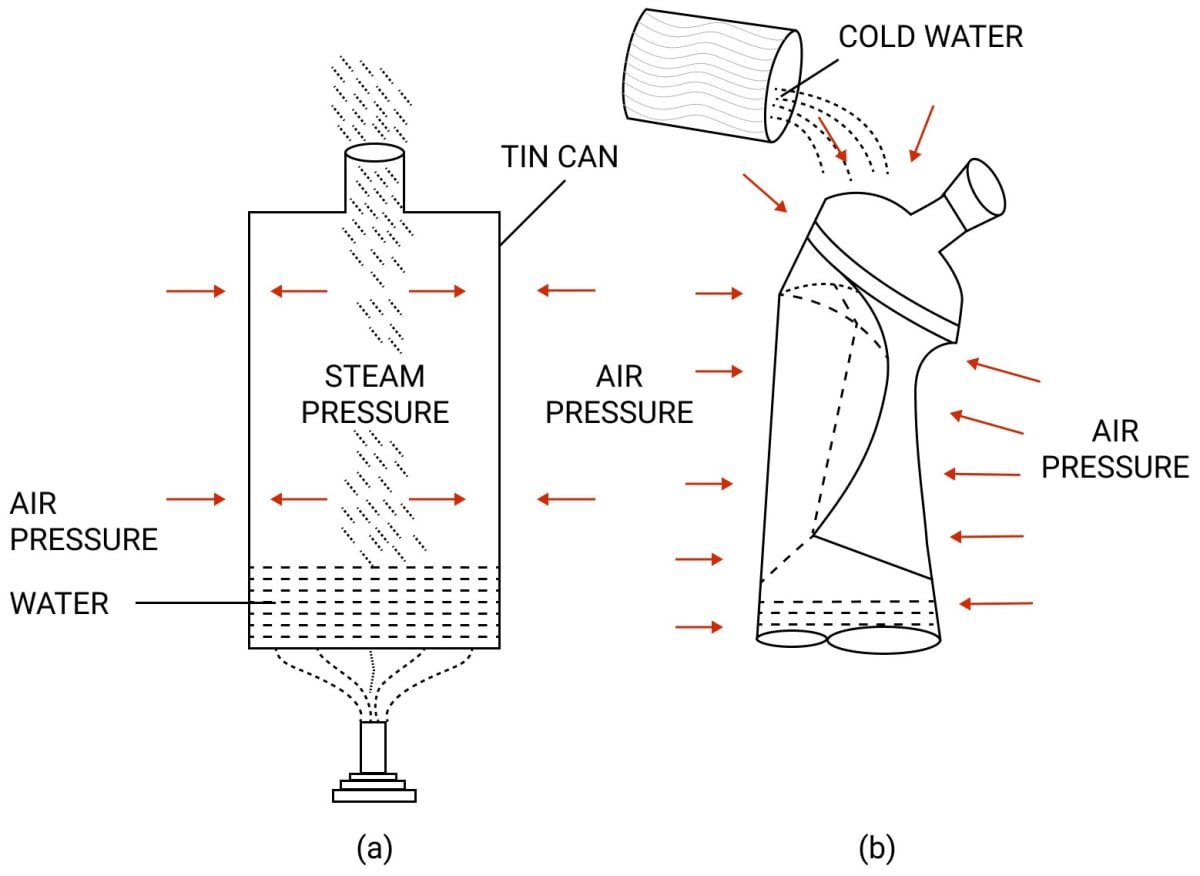#### Question 7(i)

Explain the following:

A balloon collapses when air is removed from it.

A balloon collapses when air is removed from it because as the air is released from the balloon, the pressure inside the balloon is much less than the atmospheric pressure, hence, the balloon collapses.

#### Question 7(ii)

Explain the following:

Water does not run out of a dropper unless it's rubber bulb is pressed.

Water does not run out of a dropper unless it's rubber bulb is pressed because the pressure inside the dropper is same as the atmospheric pressure when the rubber bulb is not pressed. But, when we press the rubber bulb the pressure inside the dropper increases and hence the water flows out.

#### Question 7(iii)

Explain the following:

Two holes are made in a completely filled sealed tin can to take out oil from it.

Two holes are made in a completely filled sealed tin can to take out oil from it because there is no air inside a completely filled and sealed oil can. When the can is tilted, the pressure due to the column of oil (inside the can) at the hole is much less than the atmospheric pressure outside the can, so the oil does not flow out of the hole.

But if one more hole is made at the opposite end on the top cover of the can, air from outside the can will enter in through this hole and will exert atmospheric pressure on the oil from inside along with pressure due to oil column.

This results in the increase in the pressure on oil, and so it easily flows out through the hole of the can.

#### Question 8

Why does the liquid rise in a syringe when it's piston is pulled up ?

The syringe is kept with it's opening just inside a liquid and the plunger is lowered till it's base. Now when the plunger is pulled up in the barrel (as shown in figure), there is no air inside and thus the pressure inside the barrel below the plunger is much less than the atmospheric pressure acting on the liquid surface. As a result, the atmospheric pressure forces the liquid to rise up in the syringe.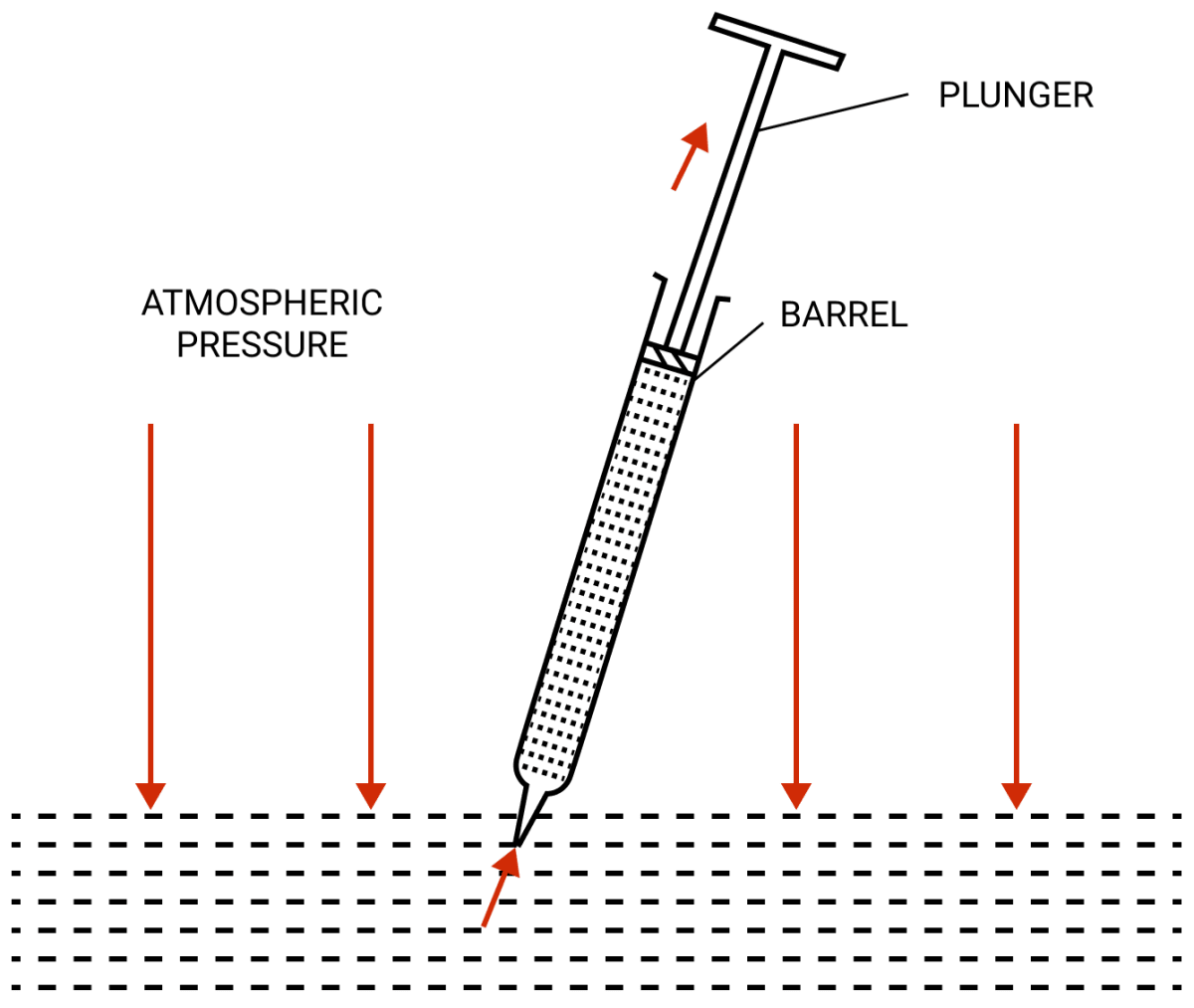#### Question 9

How is water drawn up from a well by a water pump?

In a water pump when the plunger is pulled up in the piston, there is no air inside and thus the pressure inside the siphon is much less than the atmospheric pressure acting on the liquid surface. As a result, the atmospheric pressure forces the water to rise up in the pump.

#### Question 10

A partially inflated balloon is placed inside a bell jar connected to a vacuum pump. On creating vacuum inside the bell jar, balloon gets more inflated. How does the pressure change: increase, decrease or remains same, inside the (a) bell jar and (b) balloon ?

(a) On creating vacuum inside the bell jar, the pressure inside it decreases due to the absence of air.

(b) The pressure inside the balloon remains the same as the amount of air inside the balloon does not change. The reason balloon gets more inflated on creating vacuum inside the bell jar is that due to vacuum the air surrounding the balloon has been removed so the pressure surrounding the balloon has decreased. But the air inside the ballon keeps pushing at the same pressure causing the ballon to inflate further.

#### Question 11

What is the purpose of a barometer?

The barometer is used to measure atmospheric pressure.

#### Question 12

What is a barometer? How is a simple barometer constructed?

A barometer is an instrument which is used to measure the atmospheric pressure.

A simple barometer uses mercury as the barometric liquid.

Construction — It uses a hard glass tube of about 1 m closed at one end. The tube is completely filled with pure mercury such that no air bubble remains inside the tube. The open end of tube is closed with thumb and the tube is then made upside down several times so as to force out any air bubble which might have entered in it.

The completely filled tube with it's open end closed by thumb is then inverted into a trough of mercury in such a way that the open end of tube is well immersed in mercury in a trough and the tube stands vertical as shown in figure. Now the thumb is removed. Care is taken that no air enters in the tube.

It is seen that the level of mercury in the tube falls till it's height above the level of mercury in the trough becomes h (nearly 76 cm) as shown in the diagram below.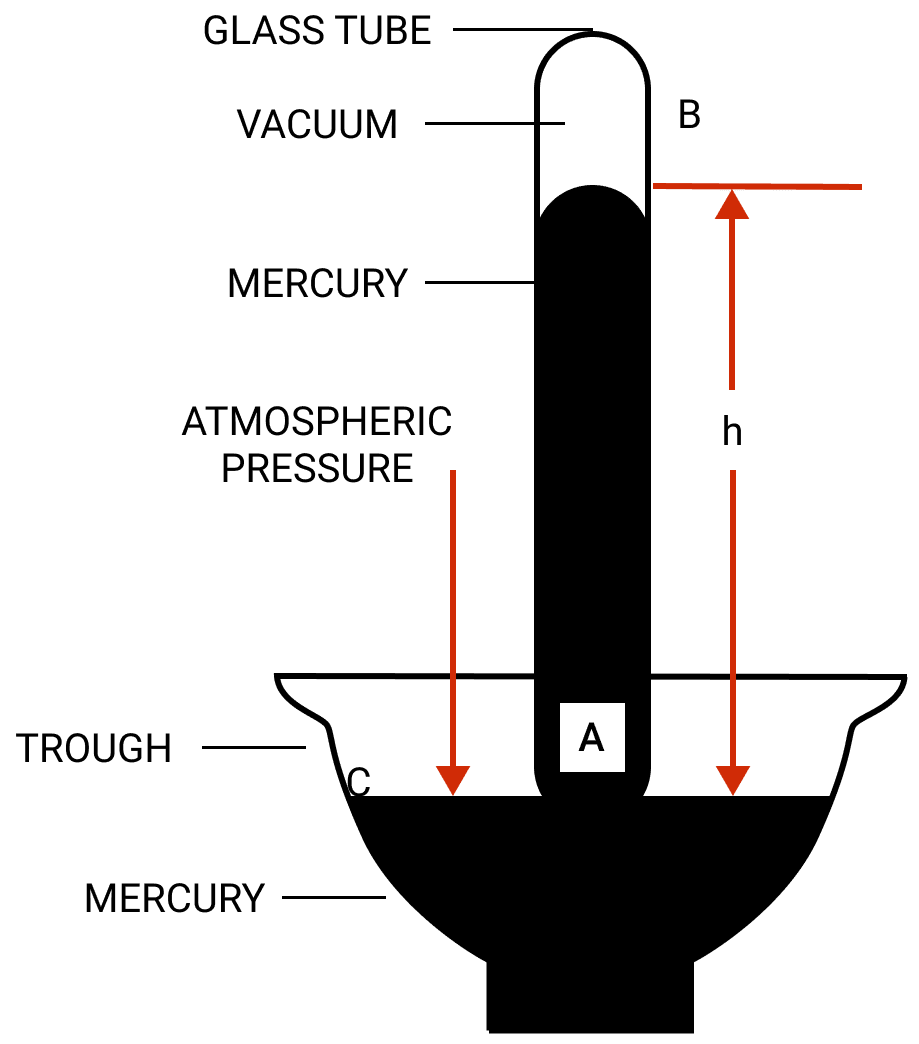#### Question 13

Explain how is the height of mercury column in the tube of a simple barometer, a measure of the atmospheric pressure.In figure, the atmospheric pressure acts at all points outside the tube (such as C) on the surface of mercury in the trough. The pressure at point A is due to the weight of the mercury column AB above it.

The mercury level in the tube becomes stationary when the pressure inside the tube at the point A which is at the level of the point C, becomes equal to that at the point C.

Thus, the vertical height of mercury column in it (i.e., AB = h) is a measure of atmospheric pressure and is called the barometric height.

The space left empty above the mercury column in the tube is called the torricellian vacuum. Ideally there should be no air in this space.

#### Question 14

Illustrate with the help of a labelled diagram of a simple barometer that the atmospheric pressure at a place is 76 cm of Hg.Vertical height of the mercury column from the mercury surface in the trough to the level in tube, is a measure of the atmospheric pressure and the barometric height at normal temperature and pressure at sea level is 0.76 m ( or 76 cm or 760 mm) of mercury.

#### Question 15

Why is the barometric height used as a unit to express the atmospheric pressure ?If the atmospheric pressure increases, the pressure at point C increases and mercury from trough flows into the tube thereby increasing the vertical height of mercury column in the tube so as to equalise pressures at points A and C.

On the other hand, when the atmospheric pressure decreases, the vertical height of the mercury column decreases to balance it.

Thus, the vertical height of the mercury column from the mercury surface in the trough to the level in the tube, is a measure of the atmospheric pressure.

#### Question 16

What is meant by the statement 'the atmospheric pressure at a place is 76 cm of Hg'? State it's value in Pa.

The statement 'the atmospheric pressure at a place is 76 cm of Hg' means that the atmospheric pressure at that place is equal to the pressure due to mercury column of height 76 cm .

The value of 76 cm of Hg = 1.013 x 105 Pa.

#### Question 17

How will you show there is vacuum above the surface of mercury in a barometer? What name is given to this vacuum ?

In an accurate barometer, the empty space above the mercury in the tube is perfect vacuum. If somehow air enters the empty space or a drop of water (or liquid) gets into the tube, it will immediately change into vapour in the vacuum space and the air (or the vapours of liquid) will exert pressure on the mercury column due to which the barometric height will decrease.

Such a barometer is a faulty barometer and it's barometric height will be less than the actual atmospheric pressure.

The name given to this space is torricellian vacuum.

#### Question 18(a)

How is the barometric height of a simple barometer affected if it's tube is pushed down into the trough of mercury?

If the tube is pushed down into the trough of mercury, the barometric height remains unaffected.

#### Question 18(b)

How is the barometric height of a simple barometer affected if it's tube is slightly tilted from vertical?

If the tube is slightly tilted from vertical, the barometric height remains unaffected.

#### Question 18(c)

How is the barometric height of a simple barometer affected if a drop of liquid is inserted inside the tube?

If a drop of liquid is inserted inside the tube, the barometric height decreases. Reason being, the drop of liquid will vapourize due to vacuum and exert pressure on the mercury column due to which the barometric height will decrease.

#### Question 19

State two uses of a barometer.

The uses of a barometer are—

1. It can be used to measure the atmospheric pressure at a place.
2. It can be used as an altimeter to measure height.

#### Question 20

Give two reasons for the use of mercury as a barometric liquid.

Uses of mercury as a barometric liquid are —

1. The vapour pressure of mercury is negligible, so the vapours in the torricellian vacuum does not affect the barometric height.
2. Mercury neither wets nor sticks to the glass tube, therefore it gives accurate reading.

#### Question 21

Give two reasons why water is not a suitable barometric liquid.

Water is not a suitable barometric liquid because —

1. The vapour pressure of water is high, so it's vapours in the vacuum space will make the reading inaccurate.
2. Water is transparent, so it's surface is not easily seen while taking the observation.

#### Question 22

Mention two demerits of a simple barometer and state how they are removed in a Fortin barometer.

The demerits of a simple barometer are —

1. There is no protection for the glass tube.
2. A scale cannot be fixed with the tube to measure the atmospheric pressure.

Fortin barometer removes these demerits in the following ways:

1. The glass tube is protected by enclosing it in a brass case.
2. For accurate measurement, a main scale alongwith a vernier scale are provided.

#### Question 23

Draw a simple labelled diagram of a Fortin barometer and state how it is used to measure the atmospheric pressure.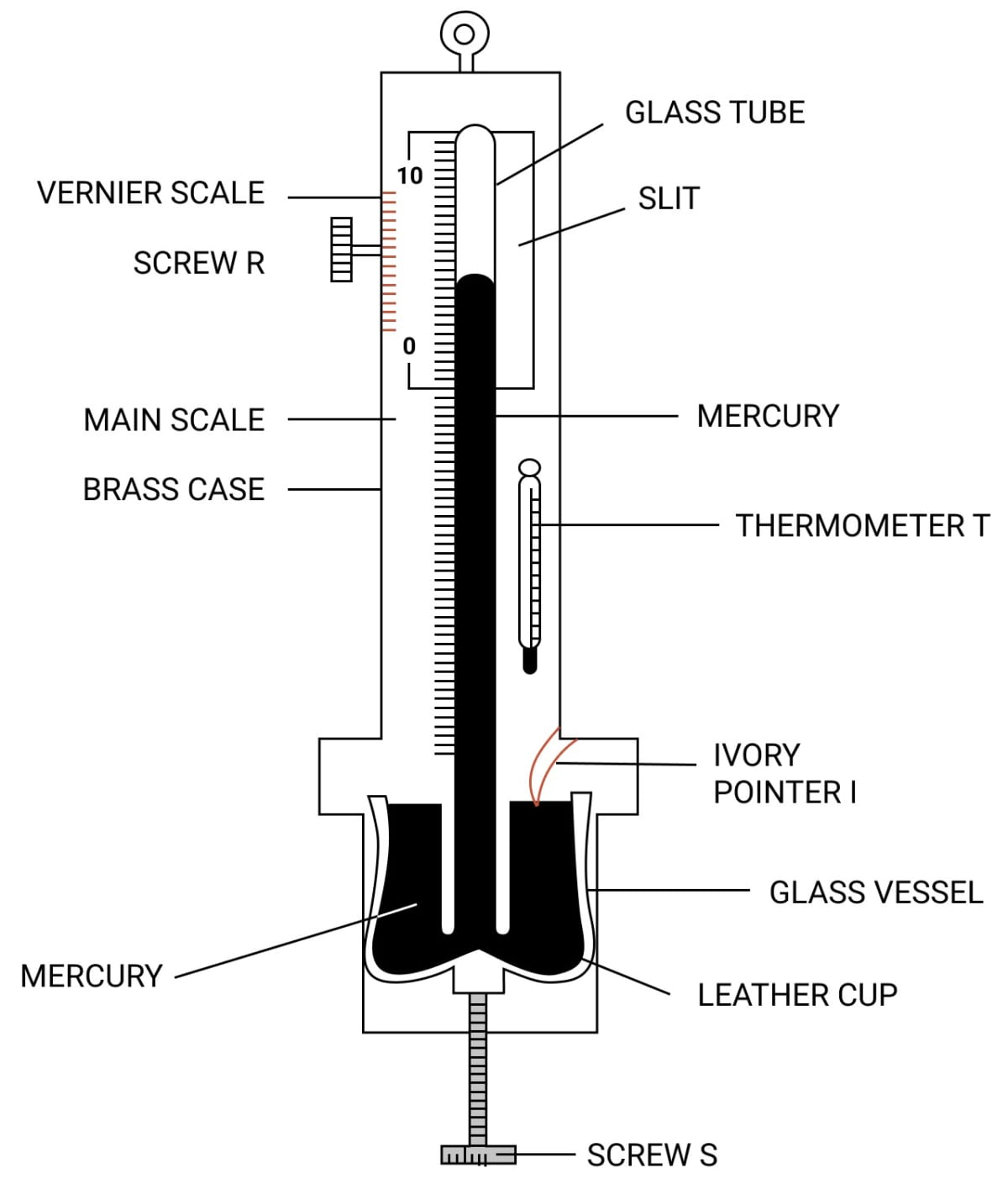To measure the atmospheric pressure, first the mercury in the leather cup is raised up or lowered down with the help of the screw S so that the mercury level in the glass vessel just touches the ivory point I. The position of mercury level in the barometer tube is noted with the help of the main scale and the vernier scale. The sum of vernier scale reading and the main scale reading gives the barometric height. The barometric height shows the atmospheric pressure.

#### Question 24

What is an aneroid barometer? Draw a neat and labelled diagram to explain its construction and working.

Aneroid barometer has no liquid. It is light and portable. It is calibrated to read directly the atmospheric pressure.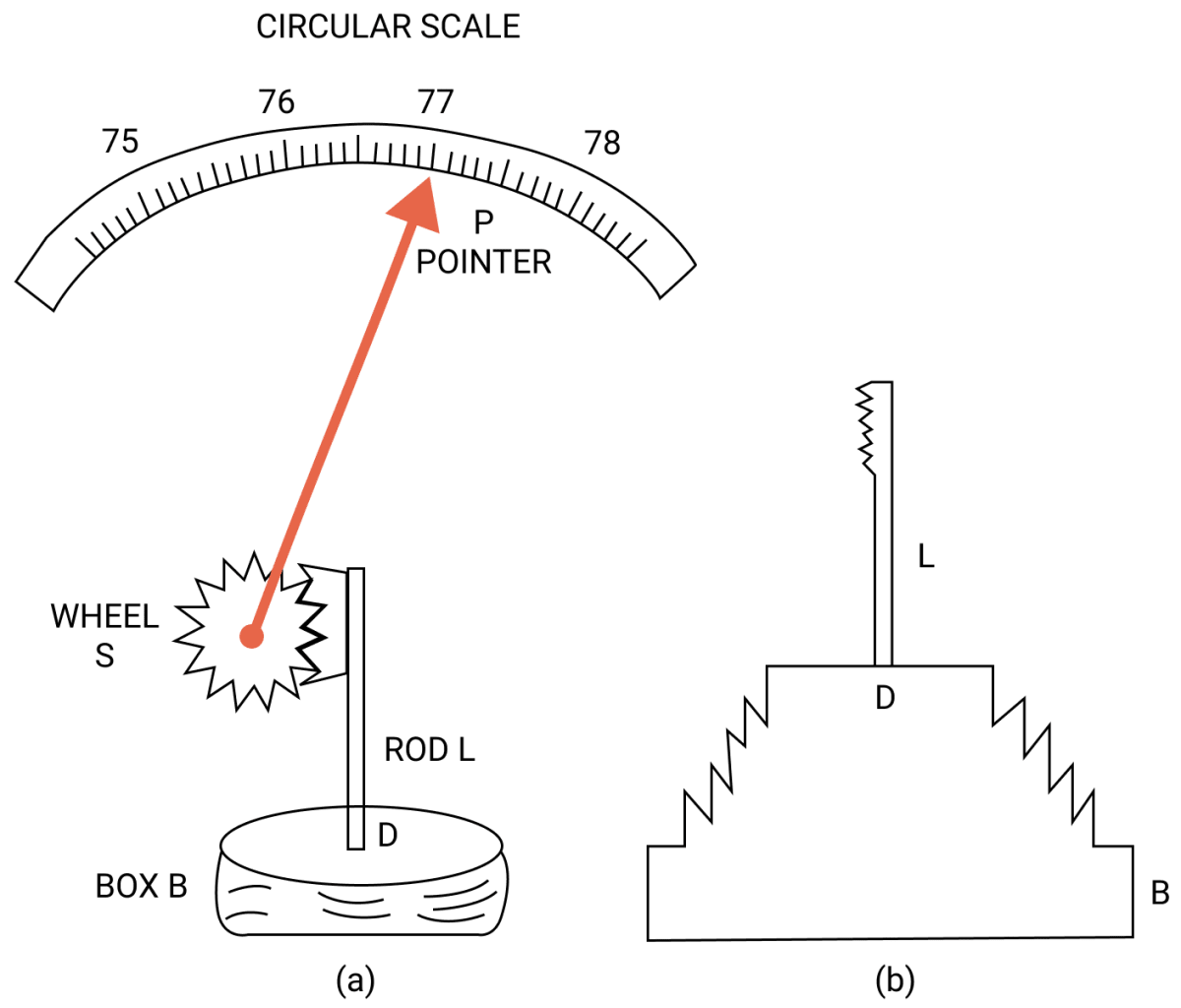Construction

The figure above shows the main parts of an aneroid barometer. It consists of a metallic box B which is partially evacuated. The top D of box is springy and is corrugated in form of a diaphragm as shown in fig (b).

At the middle of the diaphragm, there is a thin rod, toothed at it's upper end. The teeth of rod fit well into the teeth of a wheel S attached with a pointer P which can move over a circular scale.

The circular scale is graduated and is initially calibrated with a standard barometer so as to read the atmospheric pressure directly in terms of the barometric height.

Working

When the atmospheric pressure increases, it presses the diaphragm D and the rod L gets depressed. The wheel S rotates clockwise and pointer P moves to the right on the circular scale.

On the other hand, when atmospheric pressure decreases, the diaphragm D bulges out due to which the rod L moves up and the wheel S rotates anticlockwise.

Consequently, the pointer shifts to the left and the pressure is read over the calibrated scale.

#### Question 25

State two advantages of an aneroid barometer over a simple barometer.

Advantages of an aneroid barometer over a simple barometer are —

(a) Aneroid barometer is calibrated directly to read the atmospheric pressure.

(b) It has no liquid and is portable.

#### Question 26

How is the reading of a barometer affected when it is taken to (i) a mine, and (ii) a hill ?

(a) When a barometer is taken to a mine, it's reading increases because the atmospheric pressure increases with depth.

(b) When a barometer is taken to a hill, it's reading decreases because atmospheric pressure decreases with increase in altitude.

#### Question 27

How does atmospheric pressure change with altitude ? Draw an approximate graph to show this variation.

The atmosphere consists of a number of parallel air layers. Each layer experiences a pressure on it due to the thrust or weight of the air column above it. Therefore, as we go up, the height of air column above us decreases and so thrust exerted by air column also decreases, which results in the decrease of atmospheric pressure with increases in altitude.

Since the lower air layers gets compressed due to the weight (or thrust) of the upper layers, therefore, the density of air layers is more near the earth's surface and it decreases as we go higher and higher.

The decrease in density with altitude is not linear. It is rapid at low altitude (near the sea level) and is slow at higher altitude. Due to decrease in density of air with altitude, the atmospheric pressure also decreases with altitude in a non linear way.

The figure below shows the variation of atmospheric pressure with height above the sea level.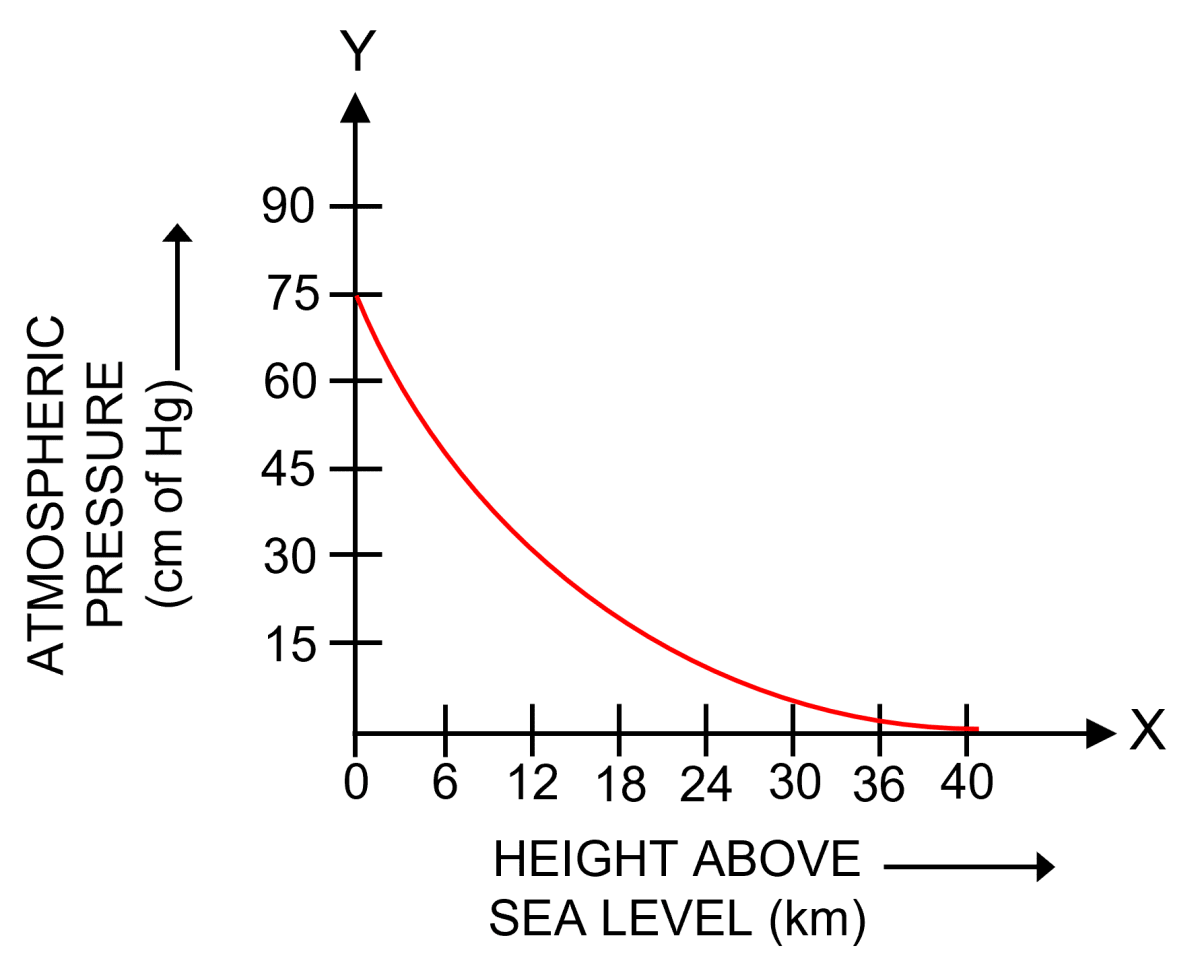#### Question 28

State two factors which affect the atmospheric pressure as we go up.

The atmospheric pressure decreases as we go up because —

1. Decrease in height of air colum which causes a linear decrease in the atmospheric pressure.
2. Decrease in density of air which causes a non-linear decrease in atmospheric pressure.

#### Question 29

Why does a fountain pen leak at a high altitude?

At high altitudes, a fountain pen leaks because normally a fountain pen filled with ink contains some air also which is at a pressure equal to the atmospheric pressure on the earths' surface. When the pen is taken at an altitude, the atmospheric pressure at this altitude is low so the excess pressure due to air inside the rubber tube forces the ink to leak out.

#### Question 30

Why does our nose start bleeding on high mountains?

Our blood pressure is slightly more than atmospheric pressure. However, at high altitude, the atmospheric pressure becomes less because the height of air column above that altitude is less than at the earth's surface. As a result, at high altitudes the blood pressure becomes much more than the atmospheric pressure and nose bleeding may occur due to excess blood pressure.

#### Question 31

What is an altimeter? State it's principle. How is it's scale calibrated ?

An altimeter is an aneroid barometer, and it is used in aircraft to measure it's altitude.

It's principle is as follows —

As atmospheric pressure decreases with the increase in height above the sea level, therefore a barometer which measures the atmospheric pressure, can be used to determine the altitude of a place above the sea level.

It's scale is calibrated in terms of height of ascent with height increasing towards left because the atmospheric pressure decreases with increase of height above the sea level.

#### Question 32

What do the following indicate in a barometer regarding weather —

(a) gradual fall in the mercury level,

(b) sudden fall in the mercury level,

(c) gradual rise in the mercury level ?

(a) If the barometric height gradually falls, it indicates that the moisture is increasing i.e., there is a possibility of rain.

(b) If the barometric height at a height suddenly falls, it means that the pressure at that place has suddenly decreased which indicates the coming of a storm or cyclone.

(c) A gradual increase in the barometric height means that the moisture in air is decreasing. This indicates the coming of dry weather.

## Multiple Choice Type

#### Question 1

The unit torr is related to the barometric height as:

1. 1 torr = 1 cm of Hg
2. 1 torr = 0.76 cm of Hg
3. 1 torr = 1 mm of Hg ✓
4. 1 torr = 1 m of Hg

1 torr = 1 mm of Hg

#### Question 2

The normal atmospheric pressure is:

1. 76 m of Hg
2. 76 cm of Hg ✓
3. 76 Pa
4. 76 N m-2

As we know that, barometric height at normal temperature and pressure at sea level is 76 cm of Hg and barometric height is the measure of the atmospheric pressure. Hence, normal atmospheric pressure = 76 cm of Hg.

#### Question 3

The atmospheric pressure at earth surface is P1 and inside mine is P2. They are related as:

1. P1 = P2
2. P1 > P2
3. P1 < P2
4. P2 = 0

Given,

P1 = atmospheric pressure at earth surface

P2 = atmospheric pressure inside mine.

As we know, when a barometer is taken to a mine, it's reading increases because the atmospheric pressure increases with depth. Hence, P1 < P2

## Numericals

#### Question 1

Convert 1 mm of Hg into pascal. Take density of Hg = 13.6 x 103 kg m-3 and g = 9.8 m s-2

As we know,

Pressure = h ρ g

Given,

ρ = 13.6 103 kg m-3

g = 9.8 m s -2

h = 1 mm = 0.001 m (As 1 m = 1000 mm = 0.001 mm)

Substituting the values in the formula above we get,

$P = (0.001) \times (13.6 \times 10^{3}) \times (9.8) \\[0.5em] \Rightarrow P = 133.28 \text{ Pa} \\[0.5em]$

Hence, 1 mm of Hg = 133.28 Pa

#### Question 2

At a given place, a mercury barometer records a pressure of 0.70 m of Hg. What would be the height of water column if mercury in barometer is replaced by water ? Take density of mercury to be 13.6 x 103 kg m-3

We know that,

Pressure = h ρ g

Given,

ρ = 13.6 x 103 kg m-3

g = 9.8 m s -2

h = 0.70 m

Substituting the values in the formula above we get,

$P = (0.70) \times (13.6 \times 10^{3}) \times (9.8) \\[0.5em] \Rightarrow P = 93.3 \times 10^{3} \text { Pa} \\[0.5em]$

Hence, P = 93.3 x 103Pa

Now, if we use a water barometer,

P = 93.3 x 103 Pa

ρ = 1 x 103 kg m-3

g = 9.8 m s -2

Substituting the values in the formula above we get,

$93.3 \times 10^{3} = (h) \times (1 \times 10^{3}) \times (9.8) \\[0.5em] h = \dfrac{93.3 \times 10^{3}}{1 \times 10^{3} \times 9.8} \\[0.5em] h = 9.52 \text { m} \\[0.5em]$

Hence, height of water colum = 9.52 m

#### Question 3

At sea level, the atmospheric pressure is 76 cm of Hg. If air pressure falls by 10 mm of Hg per 120 m of ascent, what is the height of a hill where the barometer reads 70 cm of Hg. State the assumption made by you.

Given,

Atmospheric pressure = 76 cm of Hg

Change in pressure = 76 cm of Hg - 70 cm of Hg

= 6 cm of Hg

Pressure falls by 10 mm (= 1 cm) of Hg for every 120 m of ascent,

Therefore, for 6 cm of Hg we get,

$120 \times 6 = 720 m \\[0.5em]$

Hence, the height of the hill = 720 m

The assumption made is that the atmospheric pressure falls linearly with ascent.

#### Question 4

At sea level, the atmospheric pressure is 1.04 x 105 Pa. Assuming g = 10 m s-2 and density of air to be uniform and equal to 1.3 kg m-3, find the height of the atmosphere.

We know that,

pressure = h ρ g

Given,

P = 1.04 x 105 Pa

g = 10 m s-2

density of air (ρ) = 1.3 kg m-3

Substituting the values in the formula above, we get,

$1.04 \times 10^{5} = h \times 1.3 \times 10 \\[0.5em] h = \dfrac{1.04 \times 10^{5}}{1.3 \times 10} \\[0.5em] h = 8000 \text { m} \\[0.5em]$

Hence, height of the atmosphere = 8000 m

#### Question 5

Assuming the density of air to be 1.295 kg m-3, find the fall in barometric height in mm of Hg at a height of 107 m above the sea level. Take density of mercury = 13.6 x 103 kg m-3

As we know,

decrease in pressure = h ρ g

Given,

density of air = 1.295 kg m-3

h = 107 m

Therefore,

Decrease in pressure = (107) x (1.295) x (g)    [Equation 1]

Let, decrease in mercury height = H

Therefore, decrease in barometric height = (H) x (13.6 x 103) x(g)   [Equation 2]

Equating 1 and 2 we get,

$107 \times 1.295 \times g = H \times 13.6 \times 10^{3} \times g \\[0.5em] \Rightarrow H = \dfrac{107 \times 1.295}{13.6 \times 10^{3}} \\[0.5em] \Rightarrow H = 0.0101 \text { m of Hg}$

Converting m into mm, we get

1 m = 1000 mm

Therefore, 0.0101 m = 0.0101 m x 1000 = 10.1 mm

Therefore, fall in barometric height = 10 mm of Hg.